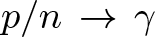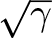Statistica Sinica 28 (2018), 2541-2564

EDGEWORTH CORRECTION FOR THE LARGEST
EIGENVALUE IN A SPIKED PCA MODEL
Jeha Yang and Iain M. Johnstone
Stanford University

Abstract: We study improved approximations to the distribution of the largest eigenvalueof the sample covariance matrix of 𝓃 zero-mean Gaussian observations in dimension p +1. We assume that one population principal component has variance 𝓁 > 1 and the remaining noise components have common variance 1. In the high-dimensional limit> 0, we study Edgeworth corrections to the limiting Gaussian distribution ofin the supercritical case 𝓁 > 1+. The skewness correction involves a quadratic polynomial, as in classical settings, but the coefficients reflect the high-dimensional structure. The methods involve Edgeworth expansions for sums of independent non-identically distributed variates obtained by conditioning on the sample noise eigenvalues, and the limiting bulk properties and fluctuations of these noise eigenvalues.

Key words and phrases: Edgeworth expansion, Roys statistic, spiked PCA model.